# MP Board Class 8th Maths Solutions Chapter 6 Square and Square Roots Ex 6.4

## MP Board Class 8th Maths Solutions Chapter 6 Square and Square Roots Ex 6.4

MP Board Class 8 Maths Chapter 6 Exercise 6.4 Question 1.
Find the square root of each of the following numbers by Division method.
(i) 2304
(ii) 4489
(iii) 3481
(iv) 529
(v) 3249
(vi) 1369
(vii) 5776
(viii) 7921
(ix) 576
(x) 1024
(xi) 3136
(xii) 900.
Solution: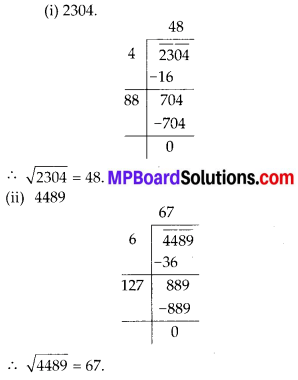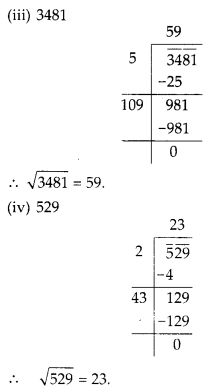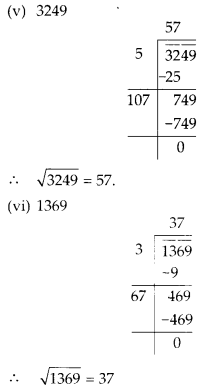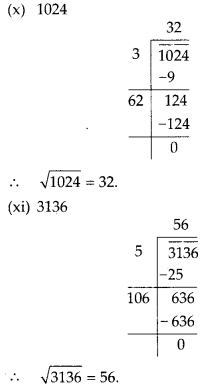Synthetic Division Calculator. The calculator will divide the polynomial by the binomial using synthetic division, with steps shown.

MP Board Class 8 Maths Chapter 6.4 Question 2.
Find the number of digits in the square root of each of the following numbers (without any calculation).
(i) 64
(ii) 144
(iii) 4489
(iv) 27225
(v) 390625
Solution:
(i) Since number of digits in 64 is 2 (= n), which is even.
Then its square root will have $$\frac{n}{2}$$ digits.
Number of digits = $$\frac{2}{2}$$ = 1.

(ii) Since the number of digits in 144 is 3 (= n), which is odd.
Then its square root will have $$\left(\frac{n+1}{2}\right)$$ digits.
Number of digits $$=\frac{3+1}{2}=\frac{4}{2}=2$$.

(iii) Since number of digits in 4489 is 4(= n), which is even.
Then its square root will have $$\frac{n}{2}$$ digits.
Number of digits = $$\frac{4}{2}$$ = 2

(iv) Since the number of digits in 27225 is 5( = n), which is odd.
Then its square root will have digits $$\left(\frac{n+1}{2}\right)$$
Number of digits $$=\frac{5+1}{2}=\frac{6}{2}=3$$

(v) Since number of digits in 390625 is 6 (= n), which is even.
Then its square root will have $$\left(\frac{n}{2}\right)$$ digits.
Number of digits = $$\frac{6}{2}$$ = 3

Online calculator that adds and subtracts roots calculator, describes all solution steps.Class 8 Maths Chapter 5 Square And Square Roots Exercise 5.4 Question 3.
Find the square root of the following decimal numbers.
(i) 2.56
(ii) 7.29
(iii) 51.84
(iv) 42.25
(v) 31.36
Solution: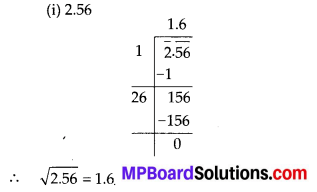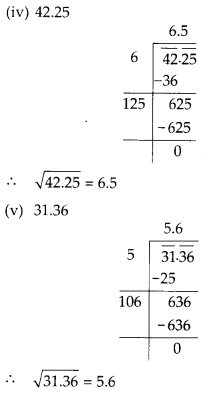Class 8 Maths Exercise 6.4 Question Number 4 Question 4.
Find the least number which must be subtracted from each of the following numbers so as to get a perfect square. Also find the square root of the perfect square so obtained.
(i) 402
(ii) 1989
(iii) 3250
(iv) 825
(v) 4000.
Solution:
(i) 402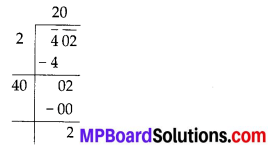Thus, if we subtract 2 from 402, we get a perfect square number whose square root is 20.

(ii) 1989Thus, if we subtract 53 from 1989, we get a perfect square number whose square root is 44.

(iii) 3250Thus, if we subtract 1 from 3250, we get a perfect square number whose square root is 57.

(iv) 825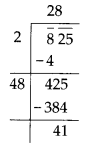Thus, if we subtract 41 from 825, we get a perfect square number whose square root is 28.

(v) 4000Thus, if we subtract 31 from 4000, we get a perfect square number whose square root is 63.Maths Class 8 Chapter 6 Exercise 6.4 Question 5.
Find the least number which must be added to each of the following numbers so as to get a perfect square. Also find the square root of the perfect square so obtained.
(i) 525
(ii) 1750
(iii) 252
(iv) 1825
(v) 6412
Solution:
(i) 525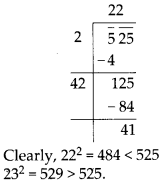Hence, the number to be added is 529 – 525 = 4 and square root of 529 is 23.

(ii) 1750Clearly, 412 = 1681 < 1750
422 = 1764 > 1750.
Hence, the number to be added is 1764 – 1750 = 14 and square root of 1764 is 42.

(iii) 252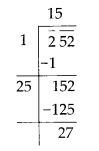Clearly, 152 = 225 < 252
162 = 256 > 252
∴ The number to be added is 256 – 252 = 4 and square root of 256 is 16.

(iv) 1825Clearly, 422 = 1764 < 1825
432 = 1849 >1825
∴ The number should be added is 1849 – 1825 = 24 and square root of 1849 is 43.

(v) 6412Clearly, 802 = 6400 < 6412
812 = 6561 > 6412
∴ The number should be added is 6561 – 6412 = 149 and square root of 6561 is 81.Exercise 6.4 Class 8 Question 5 Question 6.
Find the length of the side of a square whose area is 441 m2.
Solution:
Let the length of the side of a square is x m. Area of the square = x2 ⇒ 441 = x2
⇒ x = $$\sqrt{441}$$
⇒ x = 21
Thus, the required length of side of the square is 21 m.

Ncert Class 8 Maths Chapter 6 Exercise 6.4 Question 7.
In a right triangle ABC, ∠B = 90°.
(a) If AB = 6 cm, BC = 8 cm, find AC.
(b) If AC = 13 cm, BC = 5 cm, find AB.
Solution:
(a) AB = 6 cm, BC = 8 cm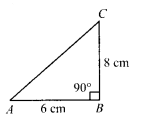By using Pythagoras theorem,
AC2 = AB2 + BC2
⇒ AC2 = (6)2 + (8)2
⇒ AC2 = 36 + 64
⇒ AC2 = 100
⇒ AC = $$\sqrt{100}$$
⇒ AC = 10 cm.

(b) AC = 13 cm, BC = 5 cm
By using Pythagoras theorem,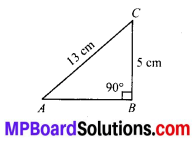AC2 = AB2 + BC2
⇒ (13)2 = AB2 + (5)2
⇒ 169 = AB2 + 25
⇒ 169 – 25 = AB2
⇒ 144 = AB2
⇒ AB = $$\sqrt{144}$$
⇒ AB = 12 cm.Class 8 Maths Square And Square Roots Exercise 6.4 Question 8.
A gardener has 1000 plants. He wants to plant these in such a way that the number of rows and the number of columns remain same. Find the minimum number of plants he needs more for this.
Solution:
Total number of plants = 1000.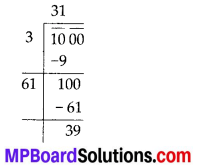Since, the plants are planted in a garden in such a way that the number of rows and the number of columns remain same.
Clearly, 312 = 961 < 1000
322 = 1024 >1000
∴ 1024 – 1000 = 24.
Thus, gardener needs 24 more plants.Class 8 Subject Maths Exercise 6.4 Question 9.
There are 500 children in a school. For a P.T. drill they have to stand in such a manner that the number of rows is equal to number of columns. How many children would be left out in this arrangement.
Solution:
Total number of children = 500.
Since the number of rows is equal to the number of colums, in which children have to stand.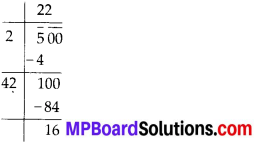Clearly, 16 children would be left out in this arrangement.